# Linear Equations, Vector Norms, and Covariance matrix

## Introduction

Machine learning and deep learning systems are built entirely on mathematical principles and notions. Understanding the underlying basis of the mathematical tenets is critical.

The basic unit of deep learning, known as a neuron, is entirely based on its mathematical idea, which entails the sum of input and weight multiplied values. Its activation functions, such as Sigmoid and ReLU, are based on mathematical theorems.

This blog will cover mathematical concepts such as linear equations, vector norms, and covariance matrices.

## Linear Equations

Many issues are formulated and solved using linear equations, which are at the heart of linear algebra. It is an equation for a straight line.

A linear equation is a mathematical expression that has two variables. A constant can be present in this equation, a linear combination of these two variables.

### Linear equation formula

Ax + By + C = 0

Where A, B are coefficients of x and y. And C is a constant.

Or

Y = bX + a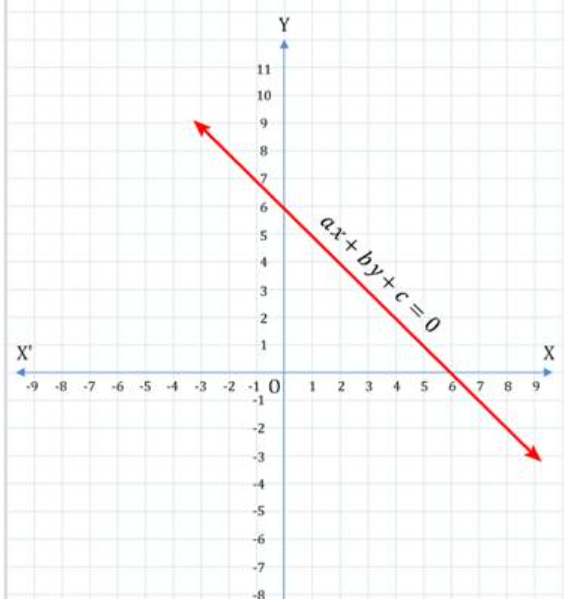Graphical Representation of Linear Equations(source)

### Linear Equation in Linear Regression

Regression is a method in machine learning for obtaining the equation for a straight line. With a certain collection of data, it tries to determine the best-fitting line. The equation of the straight line is based on the linear equation:

Y = bX + a

Where a = It is a Y-intercept and determines the point where the line crosses the Y-axis,

= It is a slope and determines the direction and degree to which the line is tilted.

### Implementation

Predict the price of a property using square feet and price as factors (machine learning model)

Reading data from a CSV file.

``````# Load the pandas libraries with the alias as 'pd.'
import pandas as pd

#(assuming this file is in the same folder)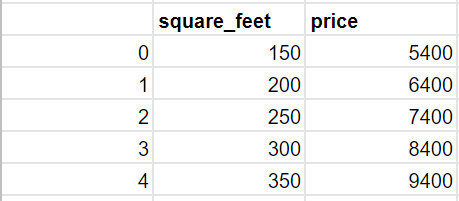House prices table.

Mean Calculation

``````def getMean(arr):
return sum(arr)/len(arr)``````

Variance Calculation

``````def getVariance(arr):
mean = getMean(arr)
meanDifferenceSquare = [(val - mean)**2 for val in arr]
return sum(meanDifferenceSquare)/(len(arr)-1)``````

Covariance Calculation

``````def getCovariance(arr1, arr2):
mean1 = getMean(arr1)
mean2 = getMean(arr2)
arr_len = len(arr1)
summation = 0.0
for j in range(0, arr_len):
summation += (arr1[i] - mean1)*(arr2[i] - mean2)

covariance = summation / (arr_len - 1)
return covariance``````

Implementing Linear Regression

``````def linearRegression(data):
X = data['square_feet']
Y = data['price']

m = len(X) # number of training

# Computing mean
squareFeetMean = getMean(X)
priceMean = getMean(Y)

# Computing variance
squareFeetVariance = getVariance(X)
priceVariance = getVariance(Y)

covarianceXY = getCovariance(X, Y)
w1 = covarianceXY
w0 = priceMean - w1 * squareFeetMean

# Linear equation for prediction
prediction = w0 - w1 * X

data['predicted price'] = prediction
return prediction``````

Invoking the linearRegression function

``linearRegression(data)``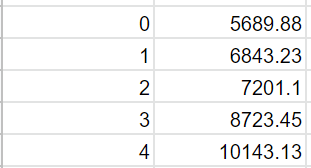Predicted Price

Linear Equation, which is used in this LinearRegression

``prediction = w0 + w1*X``

## Vector Norms

The magnitude of a vector is measured using vector norms. The size of a given variable x is represented by its norm ||x||, and the distance between two variables x and y is represented by ||x-y||.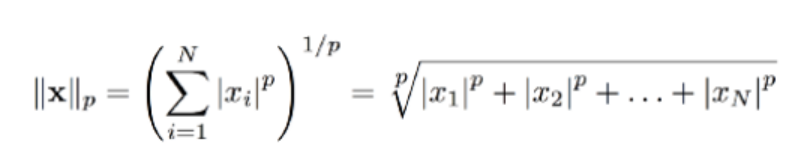Generalized equation for vector norm(source)

These are the several types of p-norms.

• Manhattan Norm (L1 norm)
• Euclidean Norm (L2 norm)

### Manhattan Norm (L1 norm)

Equation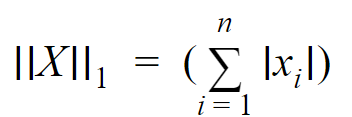Graphical Representation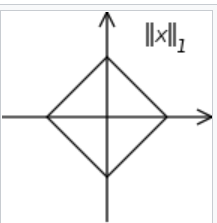### Euclidean Norm (L2 norm)

Equation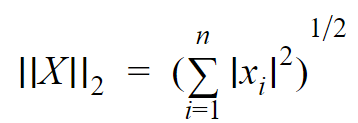Graphical Representation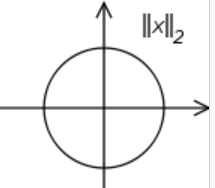### Regularization

Regularization is the act of altering the loss function to penalize specific weight on learning values. Regularization aids in the avoidance of overfitting.

It's a great addition to machine learning for the following operations:

• Handling collinearity.
• Data is filtered to remove noise.
• In order to avoid overfitting.
• To achieve good results.

The following are the most common regularisation techniques:

• L1 Regularisation (Lasso)
• L2 Regularisation (Ridge)

The formula for L1 Regularisation (Lasso)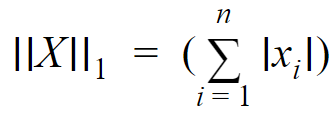The formula for L2 Regularisation (Ridge)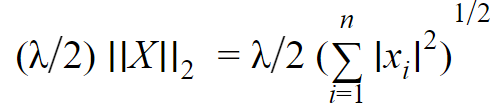Where,λ = adjusts the weight of the penalty term to control the complexity tradeoff.

## Covariance Matrix

To compute a covariance matrix, you'll need to understand the following concepts:

• Variance
• Covariance

### Variance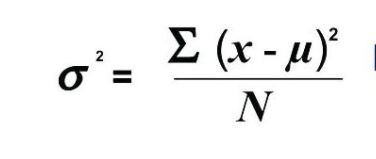The lack of exploration of the link between variables is a shortcoming of variance analysis.

### Covariance

The covariance of two random variables is used to calculate their joint variability.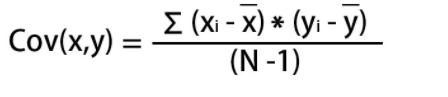### Covariance Matrix

A covariance matrix is a squared matrix that represents the correlation between two random vector elements.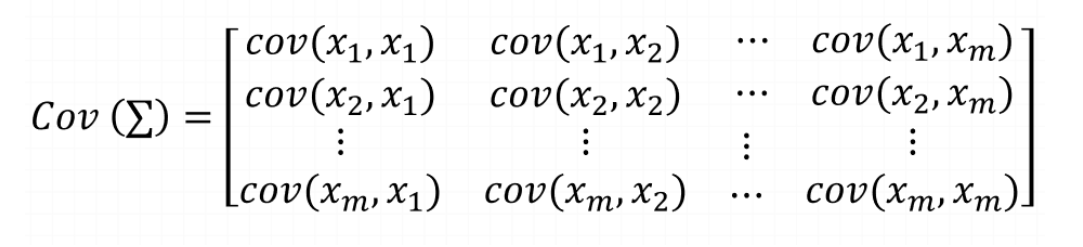Vectorized equation of covariance matrix is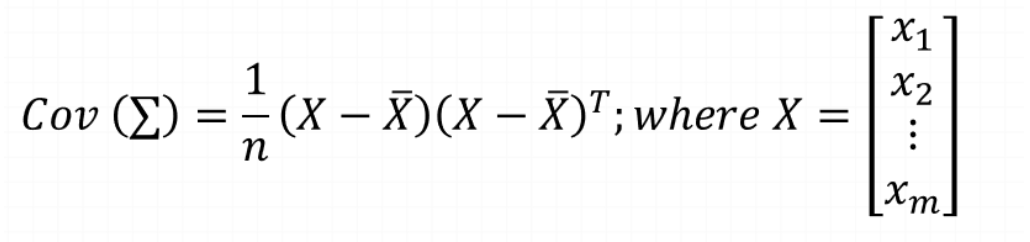## Key Takeaways

The concept of mathematics has been used to develop machine learning and deep learning. Algorithms and data computing both require a broad understanding of mathematics.

Cheers if you reached here!! You now have a better understanding of some of the essential mathematical principles that will aid in your comprehension of machine learning.

Yet learning never stops, and there is a lot more to learn. Happy Learning!!